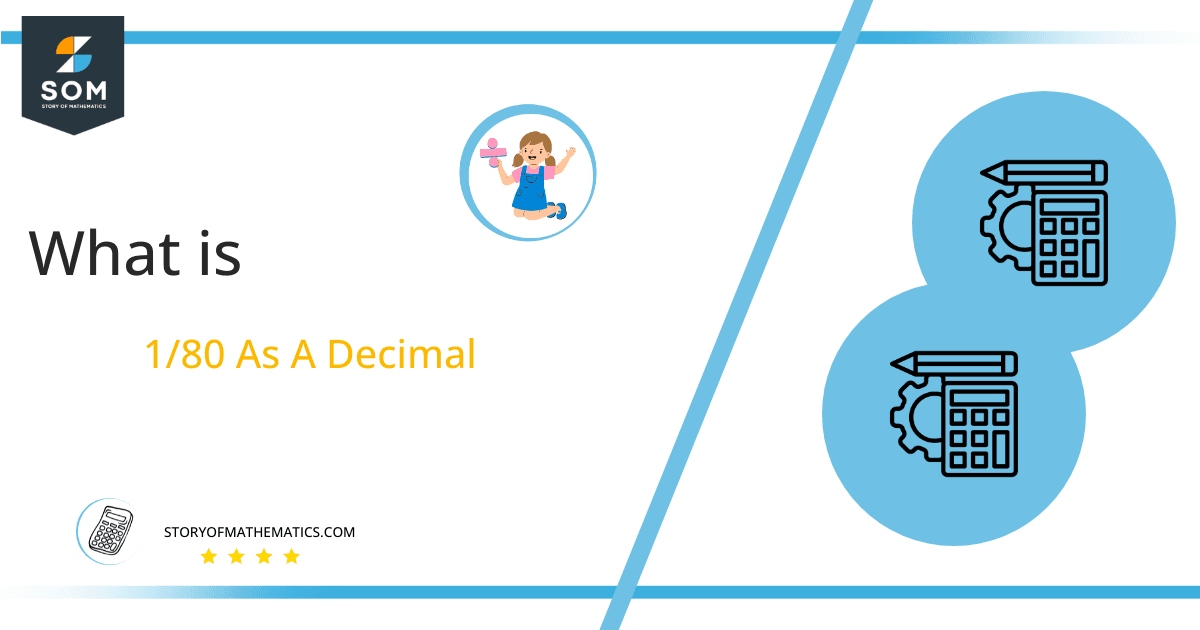# What Is 1/80 as a Decimal + Solution With Free Steps

The fraction 1/80 as a decimal is equal to 0.012.

The basic mathematical operation of division can be represented in the form of a numeral p/q, commonly known as a fraction. A fraction is simply a representation of division, and therefore all the properties of division are applicable to it. Therefore, 1/80 is equal to 1 $\boldsymbol\div$ 80.

Here, we are more interested in the division types that result in a Decimal value, as this can be expressed as a Fraction. We see fractions as a way of showing two numbers having the operation of Division between them that result in a value that lies between two Integers.Now, we introduce the method used to solve said fraction to decimal conversion, called Long Division, which we will discuss in detail moving forward. So, let’s go through the Solution of fraction 1/80.

## Solution

First, we convert the fraction components, i.e., the numerator and the denominator, and transform them into the division constituents, i.e., the Dividend and the Divisor, respectively.

This can be done as follows:

Dividend = 1

Divisor = 80

Now, we introduce the most important quantity in our division process: the Quotient. The value represents the Solution to our division and can be expressed as having the following relationship with the Division constituents:

Quotient = Dividend $\div$ Divisor = 1 $\div$ 80

This is when we go through the Long Division solution to our problem.Figure 1

## 1/80 Long Division Method

We start solving a problem using the Long Division Method by first taking apart the division’s components and comparing them. As we have 1 and 80, we can see how 1 is Smaller than 80, and to solve this division, we require that 1 be Bigger than 80.

This is done by multiplying the dividend by 10 and checking whether it is bigger than the divisor or not. If so, we calculate the Multiple of the divisor closest to the dividend and subtract it from the Dividend. This produces the Remainder, which we then use as the dividend later.

In our case, however, 1 x 10 = 10, which is still smaller than 80. Therefore, we again multiply by 10 to get 10 x 10 = 100, which is bigger than 80.

Now, we begin solving for our dividend 1, which after getting multiplied by 100 becomes 100. To indicate the double multiplication by 10, we add a decimal “.” and 0 next to it in our quotient.

We take this 100 and divide it by 80; this can be done as follows:

100 $\div$ 80 $\approx$ 1

Where:

80 x 1 = 80

This will lead to the generation of a Remainder equal to 100 – 80 = 20. Now this means we have to repeat the process by Converting the 20 into 200 and solving for that:

200 $\div$ 80 $\approx$ 2

Where:

80 x 2 = 160

This, therefore, produces another Remainder which is equal to 200 – 160 = 40. We have three decimal places now, so we stop and combine them to get the Quotient as 0.012, with a final remainder equal to 40.Images/mathematical drawings are created with GeoGebra.